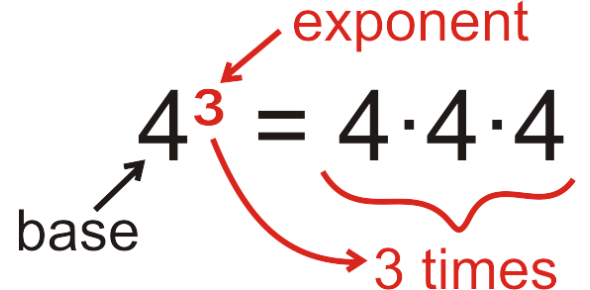# A Math Test On Exponents! Trivia Quiz

15 Questions | Attempts: 8787
ShareSettingsExponents are numbers raised to a certain number. Here we are with an exponents quiz to test your knowledge. There are different rules set aside to determine how you solve problems involving more than one exponent or an exponent and a whole number. If you are looking for a quiz to see how well you apply the set rules to get the correct solution to a problem, this test is what you need. All the best! You can share the quiz with others too.

• 1.
5+ 3  =
• 2.
3-- 7 =
• 3.
2 + 42 =
• 4.
2+ 5 =
• 5.
6+ 4 =
• 6.
103 -- 400 =
• 7.
72 + 1 =
• 8.
Which of the following numbers is prime?
• A.

14

• B.

51

• C.

47

• D.

27

• 9.
Which of the following numbers is composite?
• A.

2

• B.

19

• C.

17

• D.

15

• 10.
Which of the following numbers is neither prime nor composite?
• A.

7

• B.

1

• C.

9

• D.

11

• 11.
Which number below is square?
• A.

15

• B.

19

• C.

16

• D.

20

• 12.
Which number below is square?
• A.

100

• B.

90

• C.

50

• D.

75

• 13.
45 = 4 x 4 x 4 x 4 x 4
• A.

True

• B.

False

• 14.
2 x 2 x 2 x 5 x 5 x 5 = 23 x 52
• A.

True

• B.

False

• 15.
7 x 7 x 7 x 7 x  2 x 2 x 2 = 23 x 73
• A.

True

• B.

False

## Related TopicsBack to top
×

Wait!
Here's an interesting quiz for you.